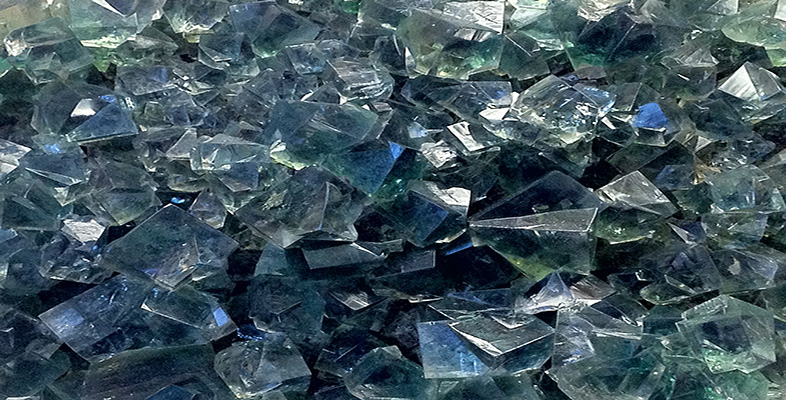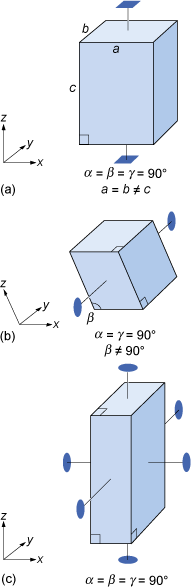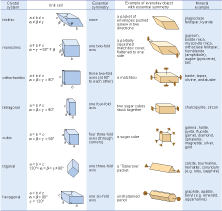Science, Maths & Technology

### Become an OU studentAn introduction to minerals and rocks under the microscope

Start this free course now. Just create an account and sign in. Enrol and complete the course for a free statement of participation or digital badge if available.

# 1.6.3 Crystal systems

Most three-dimensional lattices display some symmetry, although the symmetry elements (e.g. rotation axes and mirror planes) can be in any direction. Some arrangements of symmetry elements place special constraints on the shape of the unit cell. For example, a four-fold rotation axis requires the unit cell to have a square section at right angles to the symmetry axis (Figure 24a). Three four-fold axes at right angles to each other imply that the unit cell must be a cube, and so on.Figure 24 Symmetry and shape of some unit cells corresponding with certain rotation axes (reflection planes are not shown): (a) a four-fold rotation axis requires that the crystallographic axes are at 90° to each other, and that the unit cell has a square cross-section; (b) a single two-fold rotation axis as shown constrains two of the crystallographic axes to be at 90° to each other; (c) three two-fold rotation axes at 90° to one another imply that the three crystallographic axes are also at 90° to each other.

Crystals are classified on the basis of this three-dimensional symmetry (and hence the shapes of their unit cells) into one of seven different crystal systems (Figure 25).Figure 25 Illustration of the seven crystal systems in relation to some everyday objects, and mineral examples. Note that only the diagnostic axes of symmetry have been shown - there are many others, as well as numerous mirror planes. The trigonal system is closely related to the hexagonal system, and is sometimes considered a subsystem of it. All the minerals mentioned in this chapter (except quartz) are given, along with a few others.

The beauty of crystallography is that you do not need to see the lattice, the unit cell, or the atoms in order to deduce this symmetry. The extent of symmetry varies from the cubic system, which has the most symmetry, to triclinic, which has the least. Generally, the more symmetry a crystal has, the more constraints this places on its external shape. Crystals belonging to the cubic system tend to have equidimensional shapes, such as cubes, octahedra (with eight faces), or rather rounded-looking crystals with many faces (e.g. dodecahedra, with twelve faces). Pyrite and galena, for example, can have very simple cube-shaped crystals, which clearly indicate their underlying cubic symmetry. These same minerals may also have more complex shapes, with many more faces, but each shape still has the symmetry that places the mineral within the cubic system. The same possibility for variation within certain limits applies to other minerals in different crystal systems. Despite their complexity, by focusing on the symmetry relationships between faces, you may still be able to determine the crystal system.

Sometimes a crystal has less symmetry than first appearance suggests. For example, quartz crystals are usually prismatic, and often have a hexagonal appearance in cross-section (Digital Kit [Tip: hold Ctrl and click a link to open it in a new tab. (Hide tip)] ). At first, quartz thus appears to have a six-fold rotation axis. However, in some well-developed quartz crystals there are a number of small faces that present the same appearance only three times in a full 360° rotation, revealing that the true symmetry is less.

• Given this information, to which crystal system does quartz belong?

• The trigonal system (Figure 25), as this is the only system in which only one three-fold axis is present.

It is important to realise that conditions during the growth of a crystal often prevent some faces from developing as perfectly as they might, which results in individual crystal faces having different sizes. Some crystal faces may not be developed at all. Therefore, when looking at crystal symmetry, the angles between faces should be considered (see Figure 6), not the absolute sizes of individual faces.

## SAQ 1

• a.Study the three unit cell shapes shown in Figure 24. To which of the seven crystal systems would each belong?
• b.What would be the shape of the cross-section, at right angles to the longest side, of a crystal with a unit cell like that in Figure 24a?

## Activity 1.2 Exploring other minerals in the Digital Kit

Timing: You should allow about 30 minutes for this activity.

Use the Digital Kit to explore the properties of various minerals: calcite, orthoclase feldspar, plagioclase feldspar, galena, muscovite and quartz. Then answer the following question.

### Question 1.2.1

• a.Name one type of plagioclase feldspar.
• b.Which contains more chemical elements, pure Galena or pure calcite?
• c.What are the typical colours of orthoclase feldspar?
• d.How does the density of galena compare with all other minerals in the Digital Kit?
• e.Looking at the chemical formulae given, what is the main difference between the chemical elements present in plagioclase feldspar compared with orthoclase feldspar?
• f.Name two varieties of quartz.
• g.How do the hardness and crystal system of calcite compare with these properties of quartz?